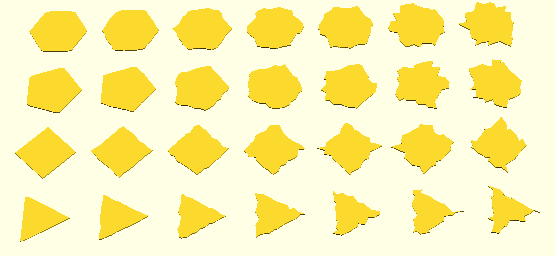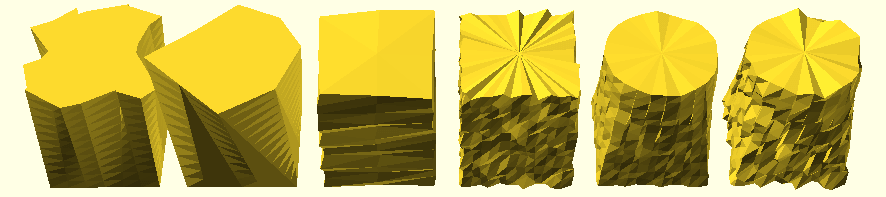This is aimed to be small OpenSCAD Library which re-implements some of its basic forms in discrete manner so vertices remain accessible in order to manipulate before being transformed into actual polyhedron for CSG operations.

State: very experimental state, not yet released due several drawbacks, code released (e.g. polygons must have same amount of segments to properly extrude, resolved)

• 2021/01/02: 0.0.6: code finally released
• 2018/12/30: 0.0.5: 3d: `dm_merge([])` merge multiple meshes into single one.
• 2018/12/26: 0.0.4:
• 3d: `dm_extrude_rotate(p,n=4,start=0,end=360)` which allows simple `dm_torus()`
• more detailed documentation and illustrations
• 2018/12/25: 0.0.3:
• 2d: added `dp_translate()`, `dp_scale()``dp_rotate()``dp_bounds()` and `dp_center()`
• 3d: added `dm_sphere()`, `dm_cylinder()` and `dm_cube()`, and `dm_translate()`, `dm_scale()``dm_rotate()``dm_bounds()` and `dm_center()` as well
• 2018/12/24: 0.0.2: `dp_morph()` as part of `dm_extrude()` uses `dp_nearest()` to find nearby point of two polygons to morph smoothly, morph examples added
• 2018/12/23: 0.0.1: Point Functions, Polygon Functions and Mesh Functions added, code not yet released

# Functions

## Discrete Point Functions

• `dpf_ellipse(r1,r2,p,a=0)`
• ​​​​`dpf_circle(r,p,a=0)`
• `dpf_circle(d,p,a=0)` must use `d=`diameter
• `dpf_rectangle(w,h,p,a=0)`
• `dpf_square(w,p,a=0)`
• `dpf_npolygon(s,p,a=0)` whereas `s` amount of sides

each function returns single 2d point, whereas

• `p` ranges between 0…1, at any resolution
• `a` is the additional rotate angle (default: 0)

## Discrete Polygon Functions

Polygon Functions use Point Functions to create an array/list of 2d points to describe a polygon; `n` amount of segments:

### Creating Polygons

• `dp_ellipse(r1,r2,n=12,a=0)`
• `dp_circle(r,n=12,a=0)`
• `dp_circle(d,n=12,a=0)` must use `d=`diameter
• all above use `dpf_ellipse()` to construct the polygon
• ellipse and circle is approximated with high count of n-sided polygon
• `dp_rectangle(w,h,n=12,a=0)`
• `dp_square(w,n=12,a=0)`
• two above use `dpf_npolygon(sqrt(2)/2,4)` and ​`dp_scale()` if required
• `dp_npolygon(r,s,n=12,a=0,start=0,end=360)` whereas `s` = amount of sides of the polygon, `s`​ > `n` * 2 for good approximation

### Manipulating Polygons

• `dp_translate(p,t)` where as `t` is a number or array [x,y]
• `dp_scale(p,t)` where as `s` is a number or array [x,y]
• `dp_rotate(p,a)`
• `dp_center(p,center=[true,true])`

further:

• ​​`dp_subdivide(p,n=2)`
• `n` amount of subdivisions per segment
• `dp_distort(a,s=1,f=1)`
• `s` amplitude of distortion
• `f` frequency of distortionN-Sided Polygon (n=3..6) with Distortion (s=0..3 with 0.5 step)
• `dp_morph(a,b,p)`
• morphs between two polygons (`a` and `b`), preferably both with same amount of segments and sufficient segments to have smooth transition
• `p` range between 0..1Morphing from square to circle and/or n-polygons in between (n=32 amount of segments)

### Query Polygons

• `[[minx,maxx],[miny,maxy]] = dp_bounds(p)`

and finally

• `dp_polygon(p)`
• creates an ordinary polygon OpenSCAD object
• Note: you no longer can access the individual vertices

## Discrete Mesh Functions

Mesh Functions use Polygon Functions to create an array with two elements:

1. list of 3d points
2. list of faces using indexes of the 3d points

### Creating Meshs

• `dm_cube(s,center=false)`
• `s` = side length or
• `s` = array of [w,d,h] or [x,y,z]
• `dm_cylinder(r,h=1,center=false,n=12)`
• `dm_cylinder(r1,r2,h=1,center=false,n=12)`
• `dm_cylinder(d,h=1,center=false)` must use `d=`diameter
• `dm_cylinder(d1,d2,h=1,center=false,n=12)` must use `d1=` and `d2=`
• `dm_sphere(r,center=false,n=12)`
• `dm_sphere(d,center=false,n=12)` must use `d=`diameterCubes, Cylinders, Cones and Sphere at different polygon resolutions

Cube and Cylinder are created by extruding `dp_rectangle` respectively `dp_circle`, whereas the Sphere is discretely done.

• `dm_torus(ri=1,ro=4,ni=48,no=48,ai=0,ao=0)`
• `ri` inner radius
• `ro` outer radius
• `ni` and `no` inner and outer n-sided polygon
• `ai` and `ao` inner and outer angle offsets
• `dm_extrude(a,b,s,h)`
• `a` & `b`: polygons
• `s` amount of steps
• `h` height (Z wise)Extruding Triangle (3-Sided Polygon) to N-Sided Polygon (s=3..12) linear and with 45 degree twist

Note: the main aim of this library was to extrude two arbitrary polygons correctly; so `dm_extrude()` is the center of this entire undertake.

• `dm_extrude_rotate(p,n=4,a=0,start=0,end=360)`
• sweep or rotate a 2d polygon, `n`-sided, used to implement `dm_torus()`

### Manipulating Meshs

• `dm_translate(m,t)` whereas `t` = number or array with [x,y,z]
• `dm_scale(m,s)` whereas `s` = number or array with [x,y,z]
• `dm_rotate(m,r)` whereas `r` = number or array with [x,y,z]
• `dm_center(m,center=[true,true,true])`
• `dm_merge(ms)` whereas `ms` is an array of meshes

and more interesting:

• `dm_distort(m,s=1.0,f=1.0)`
• distort the mesh, `s` amplitude, `f` frequencyExtruding Square to Square with top polygon or mesh distortion; Extruding Square to Circle with light and heavy distortions

### Query Meshs

• `[[minx,maxx],[miny,maxy],[minz,maxz]] = dm_bounds(m)`

and finally

• `dm_polyhedra(m);`
• creates a polyhedra which allows CSG operations
• Note: you no longer can access vertices for manipulation

# Todo

• make polygon morph (part of extruding) segment/point count neutral:
1. using `dp_morph()` which uses ​`dp_nearest(array,point)` to morph between nearest points of polygons
• disadvantage: some edges are missed where near-points don’t catch it
2. consideration: additional strategies might be required, e.g. if polygon A and B segment count difference is huge, rebalance with using `dp_subdivide()` to achieve this for smoother morphing and extruding
3. done: walking through `max(len(a),len(b))` through all points and align point indexes linearly `a[i*len(a)/max]` and `b[i*len(b)/max]`
• release code, done
• extrude along path (e.g. ​`dm_extrude_along(a,p)`)
• extrude rotate/sweep (e.g. `dm_extrude_sweep(a,r)`) done: `dm_extrude_rotate(p,n,start=0,end=360)`
• more examples, more documentation done: most functions properly illustrated with examples
• implicit meshes: define objects in implicit form (allows more natural objects with smooth edges), and transform it into meshes, see NodeJS Implicit Mesh as reference
• CSG operations on polygon (2d) or mesh (3d) level, quite challenging to do since OpenSCAD are single assignment and ​​`for()` loops must implemented recursively
• 2D: consider csg2d.js as reference
• 3D: consider csg.js as reference